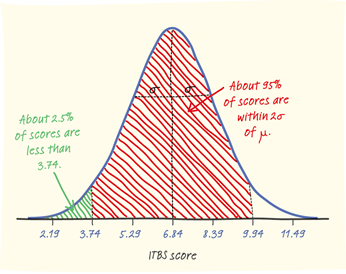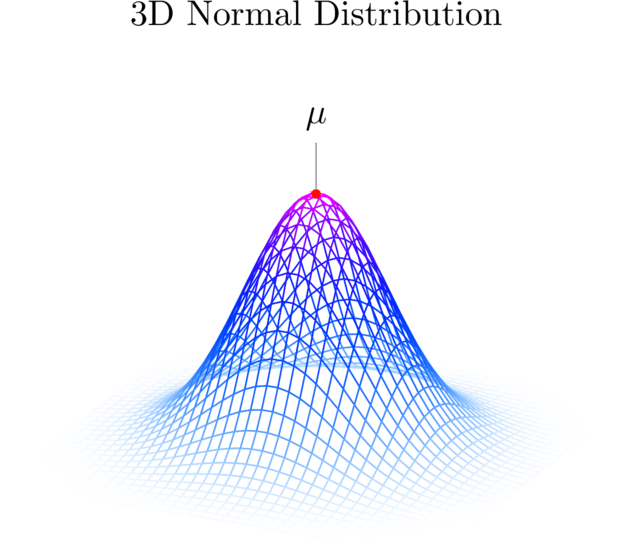# Stats Tutor in London – Statistical Inference and Data Analysis

## Statistics tutor in London for LSE, Kings, UCL, Cambridge• Describing data
• Describe how to make an inference about a population from a sample
• Select a simple random sample
• Describe different sampling methods and recognize cautions about sampling
• Distinguish between observation & experiment

In any graph of data, look for the overall pattern and for striking deviations from that pattern. You can describe the overall pattern by its shape, center, and spread. An important kind of deviation is an outlier, an individual that falls outside the overall pattern.

A distribution is symmetric if the right and left sides of the graph are approximately mirror images of each other. A distribution is skewed to the right (right-skewed) if the right side of the graph (containing the half of the observations with larger values) is much longer than the left side. It is skewed to the left (left-skewed) if the left side of the graph is much longer than the right side.

To find the mean (pronounced “x-bar”) of a set of observations, add their values and divide by the number of observations.  If the n observations are x1, x2, x3, …, xn, their mean is:The mean cannot resist the influence of extreme observations, it is not a resistant measure of center. Another common measure of center is the median

The median M is the midpoint of a distribution: half the observations are smaller and the other half are larger.

1.Arrange all observations from smallest to largest.

2.If the number of observations n is odd, the median M is the center observation in the ordered list.

3.If the number of observations n is even, the median M is the average of the two center observations in the ordered list.

### Quartiles

A useful numerical description of a distribution requires both a measure of center and a measure of spread.

To calculate the quartiles:

1) Arrange the observations in increasing order and locate the median M.

2) The first quartile Q1 is the median of the observations located to the left of the median in the ordered list.

3) The third quartile Q3 is the median of the observations located to the right of the median in the ordered list.

The interquartile range (IQR) is defined as: IQR = Q3 – Q1

Population and Sample

The population in a statistical study is the entire group of individuals about which we want information.

A sample is the part of the population from which we actually collect information. We use information from a sample to draw conclusions about the entire population.

### Random Sampling

Why should we rely on random sampling?

1.To eliminate bias in selecting samples from the list of available individuals.

2.The laws of probability allow trustworthy inference about the population.

• Results from random samples come with a margin of error that sets bounds on the size of the likely error.
• Larger random samples give better information about the population than smaller samples.

A simple random sample (SRS) of size n consists of n individuals from the population chosen in such a way that every set of n individuals has an equal chance to be the sample actually selected. In practice, people use random numbers / draws generated by computer to choose random samples. e.g. British Household Survey

### Density Curve

A density curve is a curve that:

• is always on or above the horizontal axis
• has an area of exactly 1 underneath it

A density curve describes the overall pattern of a distribution. The area under the curve and above any range of values on the horizontal axis is the proportion of all observations that fall in that range.

###Loading...Taking too long?Reload document |Open in new tab

####Loading...Taking too long?Reload document |Open in new tab

Stats Tutor Lecture Notes

Sampling Distribution

Central Limit Theorem

R_Training_for_Statistics

Other popular courses:

Get Started

## See the #1 economics mentoring platform in action

Request a demo